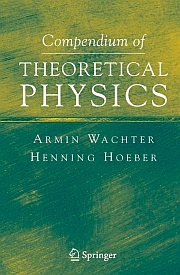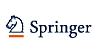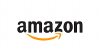# Books

Armin Wachter , Henning Hoeber

## Compendium of Theoretical PhysicsSpringer New York
ISBN: 978-0-387-25799-0

1. edition: October 2005
Hard cover, 536 pages### Description

Mechanics, Electrodynamics, Quantum Mechanics, and Statistical Mechanics and Thermodynamics comprise the canonical undergraduate curriculum of theoretical physics. In Compendium of Theoretical Physics, Armin Wachter and Henning Hoeber offer a concise, rigorous and structured overview that will be invaluable for students preparing for their qualifying examinations, readers needing a supplement to standard textbooks, and research or industrial physicists seeking a bridge between extensive textbooks and formula books.

The authors take an axiomatic-deductive approach to each topic, starting the discussion of each theory with its fundamental equations. By subsequently deriving the various physical relationships and laws in logical rather than chronological order, and by using a consistent presentation and notation throughout, they emphasize the connections between the individual theories. The reader's understanding is then reinforced with exercises, solutions and topic summaries.

Unique features:
• Every topic is reviewed axiomatically-deductively and then reinforced through exercises, solutions and summaries
• Each subchapter ends with a set of applications, making the Compendium of Theoretical Physics an ideal review of theoretical physics for physicists working in industry or research
• A Mathematical Appendix covers vector operations, integral theorems, partial differential quotients, complete function systems, Fourier analysis, Bessel functions, spherical Bessel functions, Legendre functions, Legendre polynomials and spherical harmonics

### Preface

Our book, Compendium of Theoretical Physics, contains the topics
• mechanics,
• electrodynamics,
• quantum mechanics, and
• statistical physics and thermodynamics,
which constitute the "canonical curriculum" of theoretical physics, taught at the undergraduate level at most universities. It is oriented mainly toward students of higher levels who are interested in a clearly arranged and coherent presentation of the curriculum, or who are preparing for their degree examinations. Moreover, this book is also suited as an accompanying and complementary textbook for students within the first semesters. For them, it aims to provide a useful guideline to reclassify the content of the various physics courses. Finally, physicists working in industry or research may also benefit from this overview of theoretical physics.

There are, of course, many good textbooks for the above topics (some suggestions can be found in our commented literature list). This book is therefore not to be regarded as a substitute for these books; no student can get by without an extensive study of the curriculum using other, didactically and historically well-edited presentations of theoretical physics. However, it seemed necessary to us to write this book in order to offer a complementary approach to theoretical physics, in which the composition, the structure, and, last but not least, the elegance of physical theories are emphasized and easy to recognize. We hope that this is achieved, among other things, by dispensing with historical-phenomenological explanations.

Throughout, we pursue an axiomatic-deductive approach in that we start the discussion of each theory with its fundamental equations. Subsequently, we derive the various physical relationships and laws in logical (rather than chronological) order. Our aim is to emphasize the connections between the individual theories by consistently using a standardized presentation and notation. Think, for example, of the Hamilton formalism: it constitutes a fundamental concept not only in quantum mechanics but also in statistical physics.

In the first chapter, Mechanics, we present the often overemphasized Newtonian approach to mechanics next to the Lagrange and Hamilton formulations. Each of these equivalent representations distinguishes itself by specific advantages: whereas Newton's approach is most easily accessible using the concept of force and by finding the equations of motions, only the Lagrange and Hamilton formalisms provide a suitable platform for a deeper understanding of mechanics and other theoretical concepts. For example, the Lagrange formalism is better suited to seeing the relationship between symmetries and conservation laws. Accordingly, the first three sections of this chapter deal with these three approaches and their connections in equitable fashion. Furthermore, in the section Relativistic Mechanics, we introduce the correct Lorentz tensor notation in order to ease the transition to the relativistic theory of electrodynamics, in which the disciplined use of this notation turns out to be very useful and convenient.

The advantage of our deductive method may be particularly apparent in the second chapter, Electrodynamics. In contrast to many other textbooks, we start with Maxwell's equations in their most general form. This allows us immediately to see very clearly the structure of this theory. We quickly find the general solutions to Maxwell's equations using the very important concept of gauge invariance. From this, the various laws of electrodynamics follow naturally in a clean and concise manner. For example, the solutions in empty space, or the special cases of electro- and magnetostatics, are easily deduced. Based on our results of relativistic mechanics, we apply the covariant notation to electrodynamics and discuss the Lagrange and Hamilton formalism with respect to the field theoretical character of the theory.

In contrast to the other chapters we begin Quantum Mechanics with a mathematical introduction in which some areas of linear algebra are recapitulated using Dirac's notation. In particular, the concepts of operators and eigenvalue problems are discussed. These are of fundamental importance in quantum mechanics. We then present the general structure of quantum theory, where the fundamental concepts are established and discussed in a representation-independent manner. Generally, throughout the whole chapter, we try to avoid overemphasizing a particular representation.

Similarly to mechanics, there are different approaches in statistical mechanics/thermodynamics to describe many-particle systems. First, we have the statistical ansatz that combines (quantum) mechanical laws with a statistical principle. This results in a microscopic description in form of ensemble theories. By contrast, thermodynamics is a purely phenomenological theory based on purely macroscopic experiences. A third approach is given by information theory where a system is considered from the viewpoint of lack of information. In order to highlight the inherent connections of these three concepts, we discuss all of them in our chapter Statistical Physics and Thermodynamics and show their mutual equivalence.

Throughout this book important equations and relationships are summarized in boxes containing definitions and theorems. We hope that this facilitates structured learning and makes finding fundamental results easier. Furthermore, we have arranged connected argumentations optically; in principle, the reader should always be able to recognize the end of an argument. At the end of each section we have placed a short summary as well as some applications with solutions. These applications are intended to reaffirm, and sometimes enhance, the understanding of the subject matter. Finally, in the appendix, a short compilation of important and often-used mathematical formulae are given.

It should be obvious that we make no claim of completeness. Instead, the topics of the four chapters are chosen such that, on the one hand, they contain the fundamental ideas and concepts and, on the other hand, cover the areas we have found, quite subjectively, most relevant for examinations and day-to-day work. To complement this book, we make some further literature suggestions in the appendix.

Overall, we hope that we have written a book that works as a broker between textbooks, lecture notes, and formula compilations. It would make us very happy if it helped you to better understand the concepts of theoretical physics.

Cologne and Newcastle
October 2005
Armin Wachter
Henning Hoeber